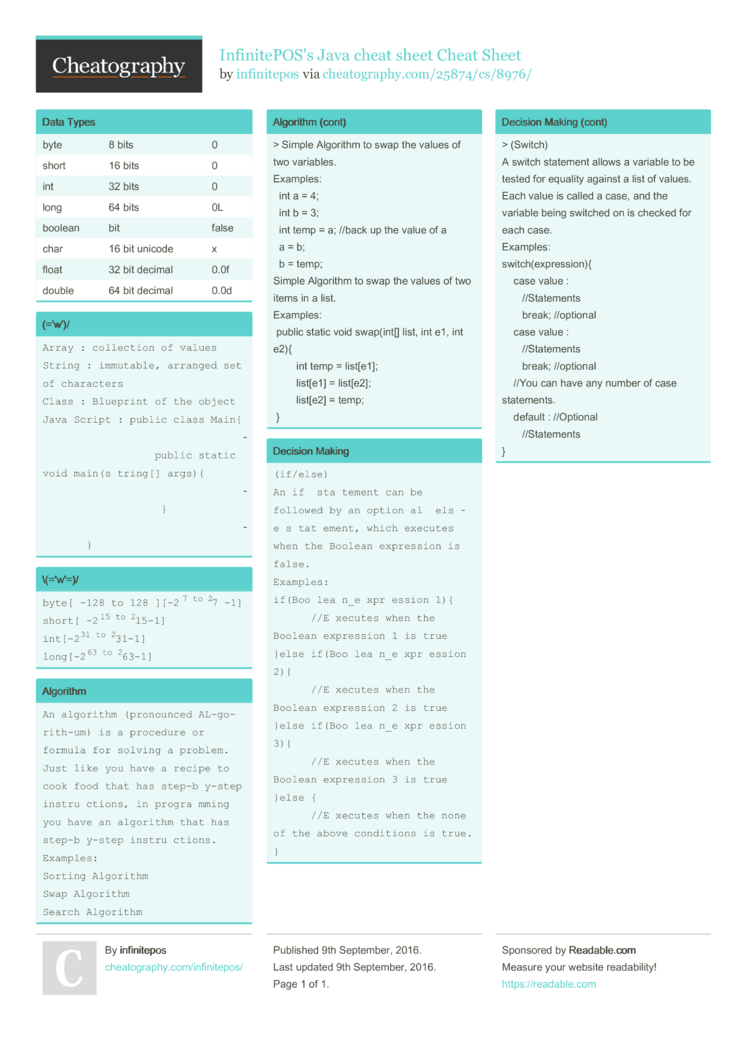# InfinitePOS's Java cheat sheet Cheat Sheet by infinitepos

### Data Types

 byte 8 bits 0 short 16 bits 0 int 32 bits 0 long 64 bits 0L boolean bit false char 16 bit unicode x float 32 bit decimal 0.0f double 64 bit decimal 0.0d

### (='w')/

 ```Array : collection of values String : immutable, arranged set of characters Class : Blueprint of the object Java Script : public class Main{                          public static void main(string[] args){                           }                     }```

### \(='w'=)/

 ```byte[ -128 to 128 ][-27 to 27 -1] short[ -215 to 215-1] int[-231 to 231-1] long[-263 to 263-1]```

### Algorithm

 ```An algorithm (pronounced AL-go-rith-um) is a procedure or formula for solving a problem. Just like you have a recipe to cook food that has step-by-step instructions, in programming you have an algorithm that has step-by-step instructions. Examples: Sorting Algorithm Swap Algorithm Search Algorithm Simple Algorithm to swap the values of two variables. Examples:   int a = 4;   int b = 3;   int temp = a; //back up the value of a   a = b;   b = temp; Simple Algorithm to swap the values of two items in a list. Examples:  public static void swap(int[] list, int e1, int e2){         int temp = list[e1];         list[e1] = list[e2];         list[e2] = temp;  }```

### Decision Making

 ```(if/else) An if statement can be followed by an optional else statement, which executes when the Boolean expression is false. Examples: if(Boolean_expression 1){    //Executes when the Boolean expression 1 is true }else if(Boolean_expression 2){    //Executes when the Boolean expression 2 is true }else if(Boolean_expression 3){    //Executes when the Boolean expression 3 is true }else {    //Executes when the none of the above conditions is true. } (Switch) A switch statement allows a variable to be tested for equality against a list of values. Each value is called a case, and the variable being switched on is checked for each case. Examples: switch(expression){     case value :        //Statements        break; //optional     case value :        //Statements        break; //optional     //You can have any number of case statements.     default : //Optional        //Statements }```1 Page
//media.cheatography.com/storage/thumb/infinitepos_infinitepos-s-java-cheat-sheet.750.jpg

PDF (recommended)